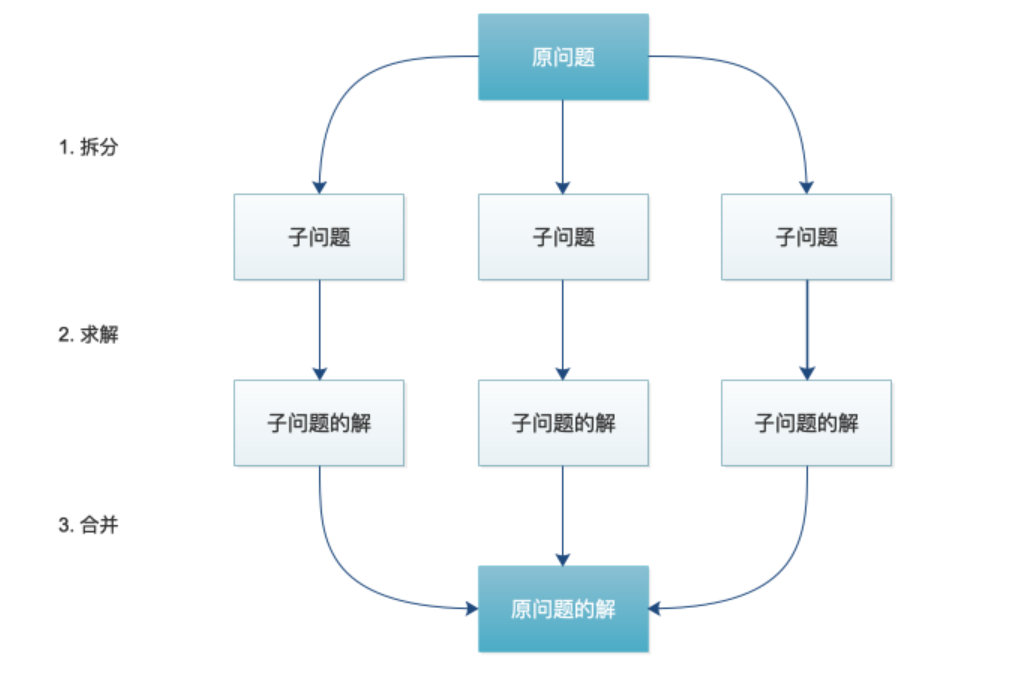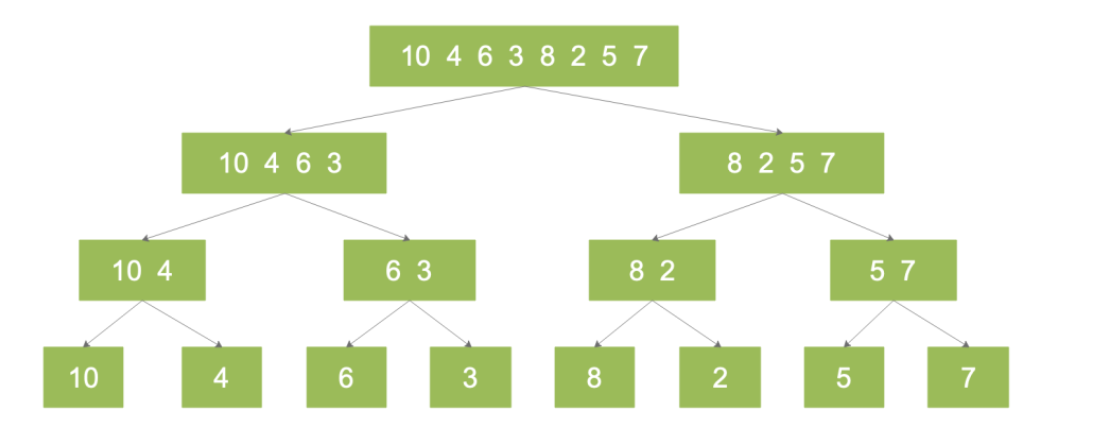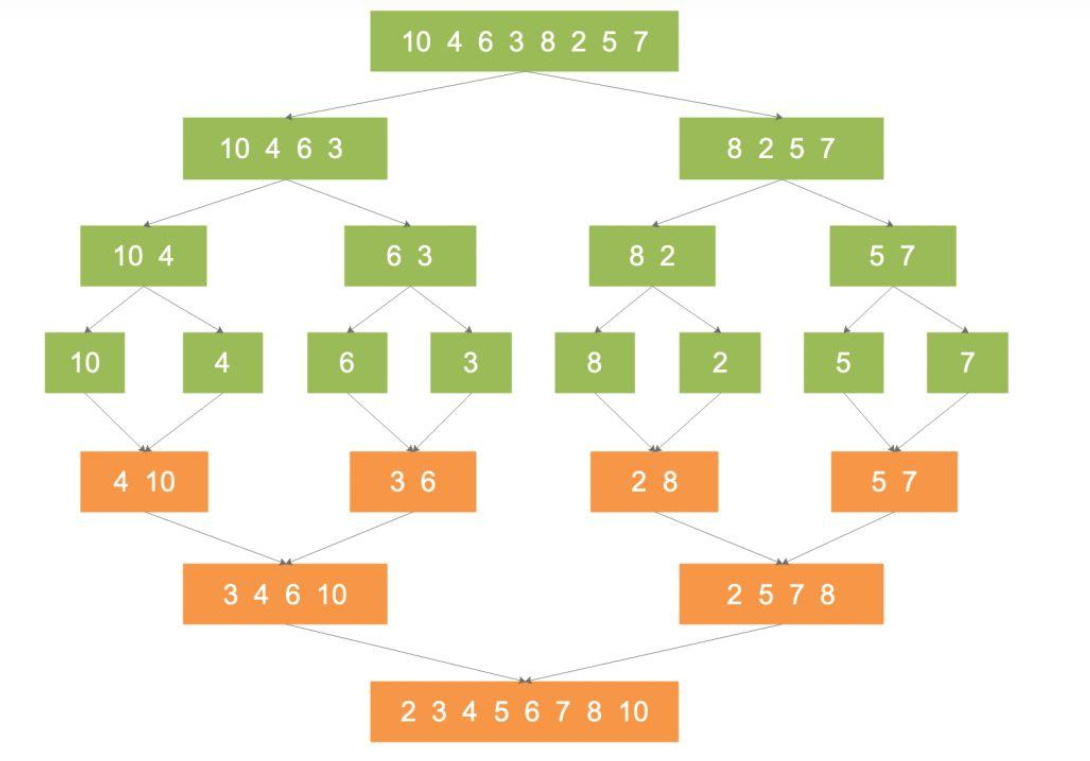## 「分治算法」三步走

1.分解：分解原问题为结构相同的子问题（即寻找子问题）
2.解决：当分解到容易求解的边界后，进行递归求解
3.合并：将子问题的解合并成原问题的解### 三步走

1.分解：将序列划分为两部分
2.解决：递归地分别对两个子序列进行归并排序
3.合并：合并排序后的两个子序列

### 举例

10, 4, 6, 3, 8, 2, 5, 710, 4 排序合并后：4, 10
6, 3 排序合并后：3, 6
8, 2 排序合并后：2, 8
5, 7 排序合并后：5, 7
……### 实现

``````def merge_sort(lst):
# 从递归中返回长度为1的序列
if len(lst) <= 1:
return lst

middle = len(lst) / 2
# 1.分解：通过不断递归，将原始序列拆分成 n 个小序列
left = merge_sort(lst[:middle])
right = merge_sort(lst[middle:])
# 进行排序与合并
return merge(left, right)

def merge(left, right):
i, j = 0, 0
result = []
# 2.解决：比较传入的两个子序列，对两个子序列进行排序
while i < len(left) and j < len(right):
if left[i] <= right[j]:
result.append(left[i])
i += 1
else:
result.append(right[j])
j += 1
# 3.合并：将排好序的子序列合并
result.extend(left[i:])
result.extend(right[j:])
return result``````

1.找到子问题并分解
2.解决子问题（递归）
3.合并子问题的解